# Surface Area Formulas

• Last Updated : 30 Jun, 2022

Surface area refers to the space occupied by the geometrical three-dimensional shape. It is denoted by the sum of the individual surfaces of the sides of a three-dimensional figure. The surface area of geometrical 3D figures can be categorized into two broad categories of total surface area and lateral surface area.

The total surface area includes the area of all the surfaces of the figure/ object(base + sides) whereas the lateral surface area of geometrical figures includes the only surface of the sides. The lateral surface area doesn’t include the area of base in its calculation. Lateral surface area or curved surface area is simply the sum of the area of sides of the geometrical figure.

Formulas below

Lateral Surface Area = Area of side faces of a geometrical figure
Total Surface Area = LSA + Area of top and base

### Formulas of Surface Area of Different Geometrical Figures

The formulas for Lateral Surface Area (LSA) and Total Surface Area(TSA) of different 3-D Geometrical Figures are given below

Surface area of Cube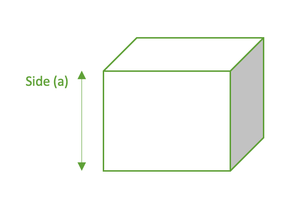Cube

• A cube is a symmetrical figure consisting of six square side faces.
• Here each face has ‘a’ units of length as sides.

LSA of cube =  4×a2
TSA of cube = 6×a2

Surface area of Cuboid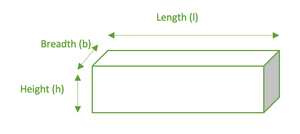Cuboid

• A cuboid is a geometrical figure consisting of six rectangular side faces.
• Here faces have ‘l’ units of length,  ‘b’ units of breadth, and ‘h’ units of height.

LSA of cube =  2×(hl + bh)
TSA of cube = 2×(hl + bh + bh)

3. Surface area of Sphere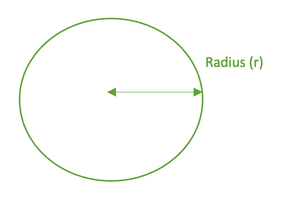Sphere

• The sphere is a 3D circular figure.
• Here, the radius has ‘r’ units of length.

LSA of sphere =  4πr2
TSA of sphere = 4πr2

Here, TSA of sphere = LSA of sphere

Surface area of the HemisphereHemisphere

• When a sphere gets sliced into two equal parts, each part is called a hemisphere.
• Here, the radius has ‘r’ units of length.

LSA of hemisphere = 2πr2
TSA of hemisphere = 3πr2

Surface Area of Cylinder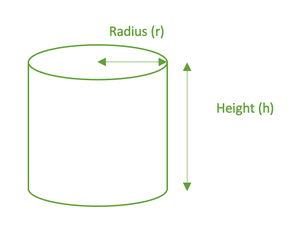Cylinder

• A cylinder is a 3D figure with two circular bases.
• Here circular base has ‘r’ units of radius and ‘h’ units of height.

LSA of cylinder = 2πrh
TSA of cylinder = 2πr2 + 2πrh

Surface area of Pyramid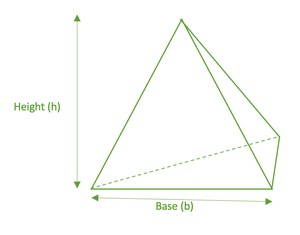Pyramid

• A pyramid is a 3D figure having triangular sides on a single given base.
• Here, the base can be a triangular, square, pentagon, or any 2-Dimensional shape.

LSA of Pyramid =  1/2 * (Perimeter of base) * Height
TSA of Pyramid = [ 1/2 * (Perimeter of base) * Height ] + Area of base

### Sample Questions

Question 1: Find the Lateral surface of a Sphere with a radius of 4 cm.

The formula of Lateral Surface Area of Sphere = 4πr2

Given r is 4cm.

= 4×3.14 × r × r

= 4 × 3.14 × 4 × 4

= 200.96 cm2

Question 2: Find the Total surface of a Hemi- Sphere with a radius of 6 cm.

Formula of Lateral Surface Area of Hemi- Sphere  = 2πr2

Given that r is 6cm

= 2 × 3.14× r ×r

= 2 * 3.14 * 6 *6

= 226.08 cm2

Question 3: Find the Total surface of a Cube with a side of 10 m.

The formula of Total Surface Area of Cube = 6a2

Given a is 10cm

= 6× a ×a

= 6× 10 × 10

= 600 m2

Question 4: Find the Lateral surface of a Cuboid with a length of 10 cm, breadth of 8 cm, and height of 6 cm.

Formula of Lateral Surface Area of Cuboid = 2×(hl + bh)

Given l is 10cm, b is 8cm, h is 6cm

= 2× (h×l + b×h)

= 2× (6×10 + 8×6)

= 2× (60 + 48)

= 2×(108)

= 216 cm2

Question 5: Find the Total surface of a Cylinder with a radius of 4 cm and height of 6 cm.

Formula of Total Surface Area of Cylinder = 2πr2 + 2πrh

Given r is 4cm and h is 6cm

= 2×3.14×r×r + 2×3.14×r×h

= 2×3.14×4×4 + 2×3.14×4×6

= 100.48 + 150.72

= 251.2 cm2

Question 6: Find the Lateral surface of a Pyramid with the base as an equilateral triangle of side 5 cm and height of 8 cm.

Formula of Lateral Surface Area of Pyramid =  1/2 × (Perimeter of base) × Height

Given h is 8cm and the base is an equilateral triangle with a side of 5cm

= 1/2 × (Perimeter of base) ×Height

= 1/2 × (5 + 5 + 5) ×Height

= 1/2 × (5 + 5 + 5) × 8

= 1/2 × (15) × 8

= 60 cm2

My Personal Notes arrow_drop_up
Recommended Articles
Page :